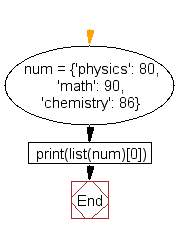﻿ Python: Access dictionary key’s element by index - w3resource# Python: Access dictionary key’s element by index

## Python Dictionary: Exercise - 55 with Solution

Write a Python program to access dictionary key's element by index.

Sample Solution:-

Python Code:

``````num = {'physics': 80, 'math': 90, 'chemistry': 86}
print(list(num))
print(list(num))
print(list(num))
```
```

Sample Output:

```physics
math
chemistry
```

Pictorial Presentation:Flowchart:## Visualize Python code execution:

The following tool visualize what the computer is doing step-by-step as it executes the said program:

Python Code Editor:

Have another way to solve this solution? Contribute your code (and comments) through Disqus.

What is the difficulty level of this exercise?

Test your Programming skills with w3resource's quiz.

﻿

## Python: Tips of the Day

How do I sort a dictionary by value?

Python 3.6+

```x = {1: 2, 3: 4, 4: 3, 2: 1, 0: 0}
{k: v for k, v in sorted(x.items(), key=lambda item: item)}
{0: 0, 2: 1, 1: 2, 4: 3, 3: 4}
```

Older Python

It is not possible to sort a dictionary, only to get a representation of a dictionary that is sorted. Dictionaries are inherently orderless, but other types, such as lists and tuples, are not. So you need an ordered data type to represent sorted values, which will be a list-probably a list of tuples.

```import operator
x = {1: 2, 3: 4, 4: 3, 2: 1, 0: 0}
sorted_x = sorted(x.items(), key=operator.itemgetter(1))
```

sorted_x will be a list of tuples sorted by the second element in each tuple. dict(sorted_x) == x.

And for those wishing to sort on keys instead of values:

```import operator
x = {1: 2, 3: 4, 4: 3, 2: 1, 0: 0}
sorted_x = sorted(x.items(), key=operator.itemgetter(0))
```

In Python3 since unpacking is not allowed  we can use

```x = {1: 2, 3: 4, 4: 3, 2: 1, 0: 0}
sorted_x = sorted(x.items(), key=lambda kv: kv)
```

If you want the output as a dict, you can use collections.OrderedDict:

```import collections

sorted_dict = collections.OrderedDict(sorted_x)
```

Ref: https://bit.ly/3dJyjpV